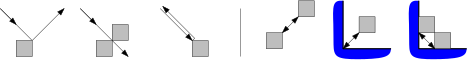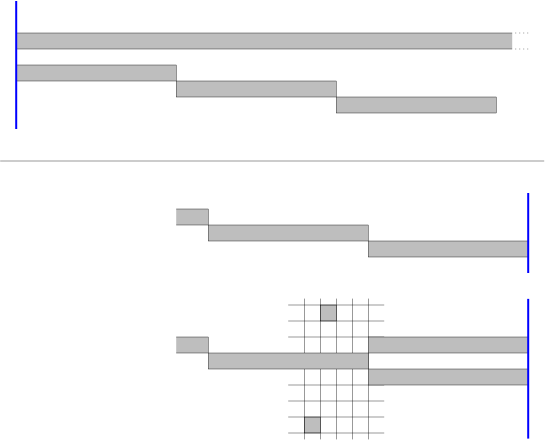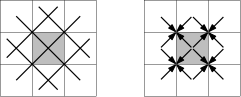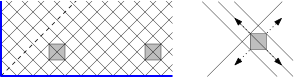# Reader Solutions to Upstart Puzzles

columnist: Dennis Shasha

# Amazing Sand Counter

No entries yet for the upstart.
• Howard Kaplan points out the following about the warm-up puzzle:

I'm writing to disagree with your proposed solution to the first Sand Counter problem, from November. You suggest that, if he can always tell how many grains of sand I removed, then that establishes that he can really count the sand.

That does not establish his ability to count the grains on a ratio scale (a scale with a real zero), only on an interval scale (a scale with an arbitrary but consistent zero). For example, if his unrevealed count is consistently off by 100 grains, he will still always report accurate differences between two separate unrevealed counts.

• My colleague Richard Cole has made the following observations on January 30, 2015.

This is a nice problem, but I think your proposed solution is not optimal. I can do 10 with six edges (assuming you allow distance 1 connections) and n with about 3/4n edges.

With solution A,B,C,D,E,F,G,H,I,J (10), use the links A --> C --> F --> J, G --> H --> I, D-->E (and distances as imposed by the solution, i.e., 2, 3, 4, 1, 1, 1,).

For the size n problem, suppose n is odd. Then do one link of length floor{(n-1)/2} from 1 to (n+1)/2 (using number names). Now do a link from (n+1)/2 + 1 to n and from n to 1. Proceed in this way, putting in longest links in each of the halves created by the first link, as well as connections to an already placed person, until n/2 people are placed. Then the remainder can be fixed by longest links without the need for connections to an already placed person.

But the solution to the 10 player problem suggests there may be still cleverer approaches.

• On February 2, 2015, Julio Marino sent me this picture, suggesting an (n/2 - 1) + n/4 solution method for n divisible by 4.
• Nagendra Gulur took some steps towards a proof with this nice discussion. Not yet fully general, but lots of good ideas there.
• Prof Don Knuth sent me the following on March 25:

Hi Dennis,

Regarding the February puzzle ("Take Your Seats"), I think two hints suffice when n=5:
B==A+1
E==C+2
and five hints when n=9:
D==A+3
F==D+2
E==B+3
H==G+1
I==H+1
(here == means congruence mod n).

But I have no idea about lower bounds or optimality.
Nice problem! -- Don

# Strategic Bullying

Richard Manion pointed out that the column shows four solutions but only three questions. There wasn't room for the fourth question, but here it is.

Sometimes, stability depends on wealth and the willingness to take risk. Suppose that we have four entities all having force 1 and all having wealth 6. If, say, E1, E2, and E3 form a coalition to defeat E4, then they divide E4's wealth, but now one of them will be the target of the other two. We say an entity E is risk-ready if it's willing to agree to an attack that might later expose E to an attack. Otherwise, we say E is risk-averse.

4. Wealth can change the calculus: suppose all entities are risk-ready and have force 1, but E1 has wealth 6, E2 has 7, E3 has 8 and E4 has 9. What will happen?

# Ice Trap

Guenter Rote (rote@inf.fu-berlin.de) vastly improved our solution (and clarified the question:) here. Here are some clarifications and assumptions for making the problem more specific:

1. A wall is a set of grid squares, i.e. a 1×n rectangle (not a line segment).
2. At each integer time t=0,1,2,…, the puck is at the center of a grid square.
3. A wall can be built or removed only at integer times.
4. The puck can hit a wall or the edge of the field at times 1/2,3/2,5/2,….
5. In boundary cases (grazing a wall, escaping through a corner, hitting a vertex head on), the puck is reflected (or not reflected) as follows:

Figure 1:On the right, there are three ways of trapping the puck with just one or two wall cells, in the middle or close to the corner of the field. Depending on the starting position of the puck, the second or third option may not be available. If it is o.k. for the wall to jump back and forth between two positions, even a single wall cell (T=1) is sufficient.

We address the grid squares as (x,y) from (0,0) (lower left corner) to (499,499), where x is the horizontal coordinate.

## A solution of the original problem in less than 1150 steps

We have a total wall length of T=500 cells at our disposal. We assume the weakest model (the third model): We hear when a wall was hit, but not which wall it was or where it was hit.

At time t=50, we build a horizontal end-to-end wall of length 500 at height y=512, and wait till the wall is hit at some time t0+½, where t0≤50+2×512−1=1073. After it is hit, we build horizontal walls of length 10 with the left endpoints at (x,y) =(0,510), (10,509), (20,508), …, (490,461), in a staggered fashion, one wall at each step t0+1, t0+2, …, t0+50.

Figure 2:If the puck is below the long wall, it will hit one of these short walls immediately after it is built. In that case, there are 12 potential locations of the puck and we can capture it quickly. We only show a solution for the last wall at y=461, which is hit at time t0+½+50. (The other cases are a bit more tricky, but more time is available to finish in these cases.) We build a facing wall and two detection bricks, as shown in Figure 2. By listening whether the puck makes one or two pauses after exiting through the corners, we can identify its position and cage it, after something like 23 additional steps, but there is certainly room for improvement in this endgame. More substantial savings are possible by placing the walls with a horizontal offset of 11 instead of 10, for example.

If no second hit occurred by time t0+½+50, we know that the puck is in the upper half. We have wasted 50 steps, but on the other hand, we know that t0≤50+2×487−1=1023. This stronger bound exactly balances the wasted time. We construct horizontal walls with their left endpoints at (x,y) =(0,564), (10,565), (20,566), …, (490,613), and the procedure runs exactly like in the lower half. The total time until the puck is confined is perhaps around 1073+50+23∼1146, with an error margin of one or two.

If it is an issue that we partially dismantle a completely built wall, we could build the initial wall of length 500 in portions of 10 bricks at a time. The case that the initial wall is put on top of the puck has not been discussed, but this is actually a lucky advantage: we know that the puck must be in a position as if it had just hit the wall, and we can immediately start the second phase without waiting.

## Catching the puck in time 83505 with one wall cell (T=1)

If left alone, the puck follows one of 501 closed loops. 499 loops are tilted rectangles, and two are the diagonals of the field. Each loop is traversed in 1000 steps. If we place a wall anywhere, it will intercept 6 of these loops, unless it touches the boundary or straddles a diagonal. If we wait 1000 time steps after placing the wall, we are sure that the puck was not on one of these loops, and we should place the wall somewhere else.

Figure 3:We put the wall on the 83 positions (3,1), (9,1), (15,1), …, (495,1) and wait 1000 units.

Figure 4:After 83 failed rounds of length 1000, at time 83001, only 3 potential tracks are left: two diagonal tracks, and one close to a diagonal. We place the wall near the center at (250,249) and wait, see Figure 4. After at most 501 steps, the puck must have hit the wall, and then it can be in one of 4 possible places and directions. After trying to block its way three times, we know precisely where it is, and we can confine it as described at the beginning.

### A lower bound of 62500

There are 500×500×4=1000000 possible states of the puck (position plus direction). The 1000000 potential initial states lead, at any particular time t, to 1000000 different states. By placing the wall somewhere, it is possible to eliminate at most 16 states, either by placing the wall on the square with the puck, or by hearing the puck hit the wall 1/2 time step later (see Figure 3, right part). This gives a lower bound of 1000000/16=62500. (The above algorithm loses a factor of 16/12 over the lower bound because it mostly leaves the wall stationary, thus wasting 4 of the 16 states that potentially could be detected. It would be interesting to discover a strategy of constantly hopping around with the wall that would come close to the lower bound.)

# Sleep No More

Georgi Guliashvili vinmesmiti@gmail.com

proposed the following: Th - Time hour
Tm - Time minute
Ah - Alarm hour
Am - Alarm minute
M - should ring that number of minutes away
---
Mh = M / 60 (Rounded down)
Mm = M % 60
F(A,T,m,R) = min( (A - (T + m) ) % R, (R - (A - (T + m) ) ) % R) Answer = F(Ah, Th, Mh, 24) + F(Am, Tm, Mm, 60)
---
Suppose we want to take a 30 minute nap and the time is 15:18 and the alarm is set to 14:50. So: Th - 15
Tm - 18
Ah - 14
Am - 50
Mh = 0
Mm = 30

F(50, 18, 30, 60) = min( (50 - (18 + 30) ) % 60, (60 - (50 - (18 + 30) ) ) % 60) = min(2, 58) = 2
F(14, 15,0,24) = min( (14 - (15 + 0) ) % 24, (24 - (14 - (15 + 0) ) ) % 24) = min(23, 1) = 1

# Chair Games

Earl Roethke earl.roethke@siemens.com pointed out that the warm-up has a two move solution:
F moves from 3 to 6
C moves from 9 to 3
which does work out nicely.

# Find Me Quickly

My colleague Ernie Davis suggested the following:

For upstart question 1, you can prove that for some graphs there is nothing better than one-player-stays-put. Suppose it is the complete graph (all pairs of nodes are connected), so that all nodes look the same. Now we can imagine that at the beginning, each player draws a map, chooses an order he's going to explore, and labels the nodes A--Z on his map. Now an adversary comes along and changes the nodes on player 1's map so that he won't meet player 2 until as late as possible; of course there is no way that player 1 can detect that anything has been changed. In the "one-player-stays-put" strategy, the adversary can at most fiddle things so that moving player hits the stationary player on move N; if both players move, the adversary can do better than that.

The algorithm for the adversary is as follows. Let Q[T] be the physical node where player 2 will be at time I; let Z[T] be the letter that player 1 has assigned for time T; and let P[U] be the adversary's mapping of player 1's letter U to a physical node. Then

Then

``````
for (U=1 .. N) {
W[U] = { Q[T] | Z[T] = U } // the places where 2 will be when 1 is
}                             // at U
renumber the U's in descending order of |W[U]|;
for (U = 1 .. N)
P[U] = any node which is not equal to P ... P[U-1]
and not an element of W[U];
``````

It is easily shown that if there are at most N different times T, then in each step of the second loop of the algorithm, the adversary has at least one option until U=N.

# Stacking the Deck

I presented this problem at the banquet of ICLP16 -- 32nd International Conference on Logic Programming. Two of the participants gave some nice solutions though not to the second upstart.

Neng-Fa Zhou wrote:

Dear Dennis,

Thank you for the entertaining puzzles. In order to show that logic programming can offer entertaining solutions, I wrote programs (in Picat) for solving the card puzzle:
http://picat-lang.org/exs/shasha_cards1.pi
http://picat-lang.org/exs/shasha_cards2.pi

Be aware that the second part of the problem, i.e., finding the minimum k, is time consuming. I hope that other LP people can offer better and more entertaining solutions.

Best regards,
Neng-Fa

Then Ingmar Dasseville wrote.

Hi,

I also found the puzzles very entertaining. I took some time to try to compete with Neng-Fa's programs.

Here is an IDP version of the shasha_cards_1: http://dtai.cs.kuleuven.be/krr/idp-ide/?src=1f49baf8fb785706cff6d63d8f98ab62

For shasha_cards_2 I took a different approach and modelled a problem which is harder for me to win (and easier to model). I take the answer for that as an upperbound. And subsequently I prove that this answer is optimal.

Instead of: for each opponent order: can we find an insertion of our cards I modelled: can we find an insertion of our cards, so that no matter what the numbers on our opponents card will be, that we will still win.
win(x) <- on(x) >= k and (forall y: k =< y =< 8 => win(x+y)).
meaning that in the model, if we find a card larger than K, we will only consider it winning, if all numbers between k and 8 lead to a win: http://dtai.cs.kuleuven.be/krr/idp-ide/?src=ca9fb22da79dd2c0b6ef62b8ff63a5ff

This problem is easily solved by our system in a very short time. Minimizing k leads to an upper bound of k=3. But of course it could be possible that our winning strategy depends on the order of our opponents cards, so we still want to prove that k = 2 is impossible

So we're left with finding an ordering of 28 cards (2-8), so that each insertion of 1's still leads to a loss. For this I wrote a Haskell program to see check a particular candidate. In this program I check whether [3,3,3,3,4,4,4,4,5,5,5,5,6,6,6,6,7,7,7,7,8,8,8,8] can be extended to a win using only four 1's. Which it can't. https://goo.gl/YrUzE3

So through the combination of the IDP and the Haskell Program we can conclude that k = 3. And both can be run in a matter of seconds. (The 3 code snippets are runnable online)

Ingmar

# Ruby Risks

Matthew Sheby pointed out that in the four different case, another possible distribution of rubies is 13, 17, 0. This by itself doesn't change the best answer which is still 12.

Also, in case it's not clear, there is no order in the boxes, so when the text says "first box", this just means one of the three boxes. Thus, for example, a possible left-to-right configuration is 17, 0, 13.

# Partitioned Peace

Richard Cole pointed out a better solution to the first challenge problem: connect the 4 R's in the middle two rows with 3 edges, and connect the top row corner R to the middle ones with a 4th edge. Do the mirror image for the B's. Swap the single isolated B on the top row with the single isolated R on the bottom row.

Chris Achenbach found another solution that uses six bridges (edges) and two swaps.
Swap (2,1) with (2,2)
Swap (2,3) with (2,4)
2 Roads form a cross: (3,1)--(4,2) and (4,1)--(3,2)
2 Roads form a cross: (3,2)--(4,3) and (4,2)--(3,3)
2 Roads form a cross: (3,3)--(4,4) and (4,3)--(3,4)
He has also created a game based on it.

# String Wars

## John Tate Solution

John Tate pointed out that the second warm-up doesn't work as written because babab matches part of Y. In fact, Y should be bbaababba.

David Abrahamson pointed out a negative answer to the upstart: X = a. Obviously, Y = b is no less expensive.

Here is a corrected version of the article with a better upstart. http://cs.nyu.edu/cs/faculty/shasha/papers/stringwarscorrected.doc

# Bounce Blockchain

Jie Wu of Temple University was the first to show that my lower bound was foolish. Even if only one site out of 20 has a certain partition, the number of sites that contain that partition can double after each time step.

Here is a corrected version with that bug fixed: http://cs.nyu.edu/cs/faculty/shasha/papers/bounceblockchainfinal_corrected.doc

# Optimal Chimes

## Mark Kaminsky Solution

Mark Kaminsky decreased the cognitive load on listeners compared to my encoding. He suggested a base-5 approach: Thus, the following table will cover the hours:
101 00:00
1011 01:00
10111 02:00
101111 03:00
1011111 04:00
1101 05:00
11011 06:00
110111 07:00
1101111 08:00
11011111 09:00
11101 10:00
111011 11:00
1110111 12:00
11101111 13:00
111011111 14:00
111101 15:00
1111011 16:00
11110111 17:00
111101111 18:00
1111011111 19:00
1111101 20:00
11111011 21:00
111110111 22:00
1111101111 23:00

As the two sets of chimes with one silence are distinctive, we need not do anything further at the hour (e.g. 22:00). For the other fifteen minute intervals, we can add:
01 x:15
011 x:30
0111 x:45

Mark calculates the time as follows: If I have figured out how you are counting the timing, the first and second pentary digits will each average about two seconds (I'll pretend that we are using 24:00 to simplify my calculations; the actual average will be slightly lower):
1 0 seconds
11 1 second
111 2 seconds
1111 3 seconds
11111 4 seconds

Then there is another two seconds for the silence between digits, so we have six seconds average to chime the hour.

The four quarter hours will take:
x:00 0 seconds
x:15 2 seconds
x:30 3 seconds
x:45 4 seconds
for an average of 2.25 seconds.

Thus, the average duration is about 8.25 seconds.

## Afroz Mohiuddin solution

```
Here is my solution for the optimal chimes puzzle:

I get an average time of 6.7seconds with my encoding for Chime Upstart, with the encoding being just a simple enumeration of all sequences following the rules sorted according to the time they take, so for k=1 the 96 sequences are:

(the code will output this when run)
Chimes: ['1', '11', '111', '101', '1111', '1011', '1101', '11111', '10111', '11011', '11101', '10101', '111111', '101111', '110111', '111011', '101011', '111101', '101101', '110101', '1111111', '1011111', '1101111', '1110111', '1010111', '1111011', '1011011', '1101011', '1111101', '1011101', '1101101', '1110101', '1010101', '11111111', '10111111', '11011111', '11101111', '10101111', '11110111', '10110111', '11010111', '11111011', '10111011', '11011011', '11101011', '10101011', '11111101', '10111101', '11011101', '11101101', '10101101', '11110101', '10110101', '11010101', '111111111', '101111111', '110111111', '111011111', '101011111', '111101111', '101101111', '110101111', '111110111', '101110111', '110110111', '111010111', '101010111', '111111011', '101111011', '110111011', '111011011', '101011011', '111101011', '101101011', '110101011', '111111101', '101111101', '110111101', '111011101', '101011101', '111101101', '101101101', '110101101', '111110101', '101110101', '110110101', '111010101', '101010101', '1111111111', '1011111111', '1101111111', '1110111111', '1010111111', '1111011111', '1011011111', '1101011111']
Chime times: [0, 1, 2, 2, 3, 3, 3, 4, 4, 4, 4, 4, 5, 5, 5, 5, 5, 5, 5, 5, 6, 6, 6, 6, 6, 6, 6, 6, 6, 6, 6, 6, 6, 7, 7, 7, 7, 7, 7, 7, 7, 7, 7, 7, 7, 7, 7, 7, 7, 7, 7, 7, 7, 7, 8, 8, 8, 8, 8, 8, 8, 8, 8, 8, 8, 8, 8, 8, 8, 8, 8, 8, 8, 8, 8, 8, 8, 8, 8, 8, 8, 8, 8, 8, 8, 8, 8, 8, 9, 9, 9, 9, 9, 9, 9, 9]
Average chime time: 6.697917

The reasoning is that, any time is equally probable, so it is useless to do something like a Huffman code - so just plain enumeration of the rule abiding sequences and sorting on time and cutting off at 96 will do.

Similarly for k=2,3,4,5 the times are:
k	t
1	6.698
2	2.958
3	2.156
4	1.750
5	1.635

giving a graph that looks like this, I'm guessing it is O(1/k^2)

My scheme increases the cognitive load on the Medievaliana citizens, but will hopefully save them some time.

```

## Matthew Sheby solution

Matthew points out that Afroz's solution assumes that a single chime sequence has a duration of zero whereas it should have one. However, he agrees with the approach. In addition, he has python code for up to 96 different chime codes.

## Nagendra and Ananya Gd

```
I think we found a slightly better solution than Mohiuddin's.

Using the scoring scheme of 1 chime == 1 second and gap == 2 seconds, we evaluated the average duration of 4 different schemes:

- a basic scheme that picks up the first 96 unique binary codes that satisfy the requirements
- Mohiuddin's published solution
- a variant of Mohiuddin's where I replaced the decimal value 341 with the value 2047.

These schemes respectively have average durations of 9.5, 10.5, 9.333333, and 9.3125 seconds.

Mohiuddin's algorithm appears correct except perhaps the scoring scheme being different makes a slight difference or they cut off their algorithm a little too early and did not let it discover 2047 as a better selection.

The better solution in binary is:
['1', '11', '111', '101', '1111', '1011', '1101', '11111', '10111', '11011', '11101', '10101', '111111', '101111', '110111', '111011', '101011', '111101', '101101', '110101', '1111111', '1011111', '1101111', '1110111', '1010111', '1111011', '1011011', '1101011', '1111101', '1011101', '1101101', '1110101', '1010101', '11111111', '10111111', '11011111', '11101111', '10101111', '11110111', '10110111', '11010111', '11111011', '10111011', '11011011', '11101011', '10101011', '11111101', '10111101', '11011101', '11101101', '10101101', '11110101', '10110101', '11010101', '111111111', '101111111', '110111111', '111011111', '101011111', '111101111', '101101111', '110101111', '111110111', '101110111', '110110111', '111010111', '101010111', '111111011', '101111011', '110111011', '111011011', '101011011', '111101011', '101101011', '110101011', '111111101', '101111101', '110111101', '111011101', '101011101', '111101101', '101101101', '110101101', '111110101', '101110101', '110110101', '111010101', '11111111111', '1111111111', '1011111111', '1101111111', '1110111111', '1010111111', '1111011111', '1011011111', '1101011111']

```

Here is their code.

```
I said "we" above as my daughter got quite excited to learn "binary numbers" and enthusiastically participated in the whole exercise. She is 12.

Best Regards
Nagendra and Ananya
```